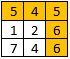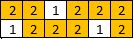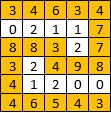##### Welcome to Subscribe On Youtube

Formatted question description: https://leetcode.ca/all/1102.html

# 1102. Path With Maximum Minimum Value

Medium

## Description

Given a matrix of integers A with R rows and C columns, find the maximum score of a path starting at [0,0] and ending at [R-1,C-1].

The score of a path is the minimum value in that path. For example, the value of the path 8 → 4 → 5 → 9 is 4.

A path moves some number of times from one visited cell to any neighbouring unvisited cell in one of the 4 cardinal directions (north, east, west, south).

Example 1:Input: [[5,4,5],[1,2,6],[7,4,6]]

Output: 4

Explanation: The path with the maximum score is highlighted in yellow.

Example 2:Input: [[2,2,1,2,2,2],[1,2,2,2,1,2]]

Output: 2

Example 3:Input: [[3,4,6,3,4],[0,2,1,1,7],[8,8,3,2,7],[3,2,4,9,8],[4,1,2,0,0],[4,6,5,4,3]]

Output: 3

Note:

1. 1 <= R, C <= 100
2. 0 <= A[i][j] <= 10^9

## Solution

For each cell in the matrix, store its row, column and score in the path, where score in the path is the minimum value in the path. Use a priority queue to store the cells, where the cell with the maximum score is polled first. Each time poll a cell from the priority queue, and update its adjacent cells’ scores. Make sure that each cell is visited only once. Since a priority queue is used, it is guaranteed that each cell’s score is the maximum possible score. Finally, return the score at the bottom right corner.

• class Solution {
public int maximumMinimumPath(int[][] A) {
final int WHITE = 0;
final int GRAY = 1;
final int BLACK = 2;
int rows = A.length, columns = A.length;
int[][] colors = new int[rows][columns];
int[][] values = new int[rows][columns];
int[][] directions = { {-1, 0}, {1, 0}, {0, -1}, {0, 1} };
PriorityQueue<int[]> priorityQueue = new PriorityQueue<int[]>(new Comparator<int[]>() {
public int compare(int[] array1, int[] array2) {
if (array1 != array2)
return array2 - array1;
else if (array1 != array2)
return array1 - array2;
else
return array1 - array2;
}
});
colors = GRAY;
values = A;
priorityQueue.offer(new int[]{0, 0, values});
while (!priorityQueue.isEmpty()) {
int[] cell = priorityQueue.poll();
int row = cell, column = cell, value = cell;
for (int[] direction : directions) {
int newRow = row + direction, newColumn = column + direction;
if (newRow >= 0 && newRow < rows && newColumn >= 0 && newColumn < columns && colors[newRow][newColumn] == WHITE) {
colors[newRow][newColumn] = GRAY;
values[newRow][newColumn] = Math.min(value, A[newRow][newColumn]);
priorityQueue.offer(new int[]{newRow, newColumn, values[newRow][newColumn]});
}
}
colors[row][column] = BLACK;
}
return values[rows - 1][columns - 1];
}
}

• // OJ: https://leetcode.com/problems/path-with-maximum-minimum-value/
// Time: O(MNlog(MN))
// Space: O(MN)
class Solution {
typedef array<int, 3> T;
public:
int maximumMinimumPath(vector<vector<int>>& A) {
int M = A.size(), N = A.size(), dirs = { {0,1},{0,-1},{1,0},{-1,0} };
priority_queue<T> pq;
pq.push({A,0,0});
A = -1;
while (pq.size()) {
auto [score, x, y] = pq.top();
pq.pop();
if (x == M - 1 && y == N - 1) return score;
for (auto &[dx, dy] : dirs) {
int a = x + dx, b = y + dy;
if (a < 0 || a >= M || b < 0 || b >= N || A[a][b] == -1) continue;
pq.push({ min(score, A[a][b]), a, b });
A[a][b] = -1;
}
}
return -1;
}
};

• class Solution:
def maximumMinimumPath(self, grid: List[List[int]]) -> int:
def find(x):
if p[x] != x:
p[x] = find(p[x])
return p[x]

m, n = len(grid), len(grid)
p = list(range(m * n))
ans = min(grid, grid[-1][-1])
vis = {(0, 0), (m - 1, n - 1)}
scores = [[grid[i][j], i, j] for i in range(m) for j in range(n)]
scores.sort()
while find(0) != find(m * n - 1):
score, i, j = scores.pop()
ans = min(ans, score)